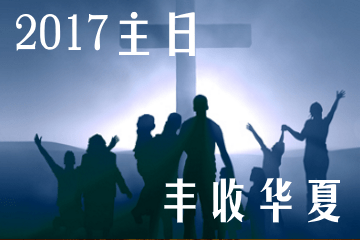# 华夏2017主日Free## 2017/10/08 豐收華夏教會主日現場全球網絡直播## 大陆视频### Course Features

• Lectures 53
• Quizzes 0
• Duration 20 hours
• Skill level All levels
• Language English
• Students 0
• Assessments Self
• Lecture1.1
1h0m
• Lecture1.2
1h0m
• Lecture1.3
1h0m
• Lecture1.4
1h0m
• Lecture1.5
1h0m
• Lecture1.6
1h0m
• Lecture1.7
1h0m
• Lecture1.8
1h0m
• Lecture1.9
1h0m
• Lecture1.10
1h0m
• Lecture1.11
1h0m
• Lecture1.12
1h0m
• Lecture1.13
1h0m
• Lecture1.14
1h0m
• Lecture1.15
1h0m
• Lecture1.16
1h0m
• Lecture1.17
1h0m
• Lecture1.18
1h0m
• Lecture1.19
1h0m
• Lecture1.20
1h0m
• Lecture1.21
1h0m
• Lecture1.22
1h0m
• Lecture1.23
1h0m
• Lecture1.24
1h0m
• Lecture1.25
1h0m
• Lecture1.26
1h0m
• Lecture1.27
1h0m
• Lecture1.28
1h0m
• Lecture1.29
1h0m
• Lecture1.30
1h0m
• Lecture1.31
1h0m
• Lecture1.32
1h0m
• Lecture1.33
1h0m
• Lecture1.34
1h0m
• Lecture1.35
1h0m
• Lecture1.36
1h0m
• Lecture1.37
1h0m
• Lecture1.38
1h0m
• Lecture1.39
1h0m
• Lecture1.40
1h0m
• Lecture1.41
1h0m
• Lecture1.42
1h0m
• Lecture1.43
1h0m
• Lecture1.44
1h0m
• Lecture1.45
1h0m
• Lecture1.46
1h0m
• Lecture1.47
1h0m
• Lecture1.48
1h0m
• Lecture1.49
1h0m
• Lecture1.50
1h0m
• Lecture1.51
1h0m
• Lecture1.52
1h0m
• Lecture1.53
1h0m## 帖撒罗尼迦前书

0
Free## 歌罗西书

0
Free0
Free